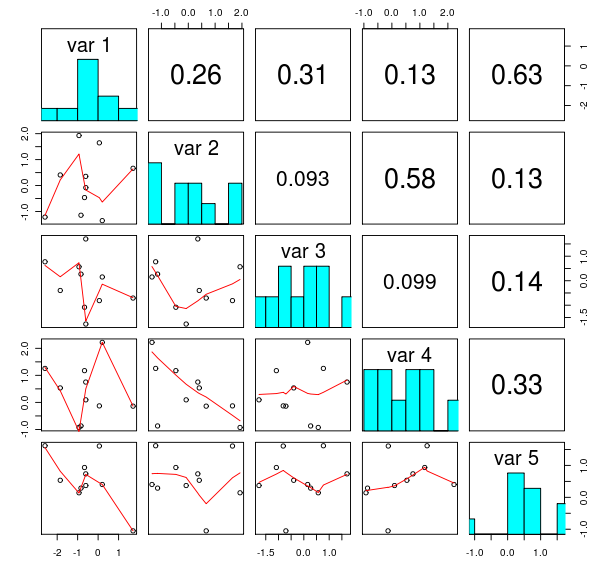# matplotlib analog of R's `pairs`

## Question:

R has a useful function `pairs` that provides nice matrix of plots of pairwise connections between variables in a data set. The resulting plot looks similar to the following figure, copied from this blog post:Is there any ready to use function based on python’s matplolib? I have searched its gallery, but couldn’t find anything that resembles what I need. Technically, this should be a simple task, but proper handling of all the possible cases, labels, titles, etc is very tedious.

UPDATE see below my answer with a quick and dirty approximation.

As far as I know, there’s not a ready-to-use function like that.

Quick and dirty approximation to my needs:

``````def pair(data, labels=None):
""" Generate something similar to R `pair` """

nVariables = data.shape
if labels is None:
labels = ['var%d'%i for i in range(nVariables)]
fig = pl.figure()
for i in range(nVariables):
for j in range(nVariables):
nSub = i * nVariables + j + 1
if i == j:
ax.hist(data[:,i])
ax.set_title(labels[i])
else:
ax.plot(data[:,i], data[:,j], '.k')

return fig
``````

The code above is hereby released into the public domain

`Pandas` has a built in function `scatter_matrix` (source code) which is something like this.

``````import numpy as np
import pandas as pd
import matplotlib.pyplot as plt

df = pd.DataFrame(np.random.randn(1000, 4), columns=['A','B','C','D'])
axes = pd.tools.plotting.scatter_matrix(df, alpha=0.2)
plt.tight_layout()
plt.savefig('scatter_matrix.png')
``````However it is `pandas` specific (but could be used as a starting point).

There are some more `R` like plots in pandas. Have a look at the docs.

The `subplots` function in recent versions of matplotlib (at least 1.4) makes this a little bit easier:

``````def pairs(data, names):
"Quick&dirty scatterplot matrix"
d = len(data)
fig, axes = plt.subplots(nrows=d, ncols=d, sharex='col', sharey='row')
for i in range(d):
for j in range(d):
ax = axes[i,j]
if i == j:
ax.text(0.5, 0.5, names[i], transform=ax.transAxes,
horizontalalignment='center', verticalalignment='center',
fontsize=16)
else:
ax.scatter(data[j], data[i], s=10)
``````

In recent versions(after 0.23.3 or maybe in few version earlier too) you can use:

``````In : from pandas.plotting import scatter_matrix

In : df = pd.DataFrame(np.random.randn(1000, 4), columns=['a', 'b', 'c', 'd'])

In : scatter_matrix(df, alpha=0.2, figsize=(6, 6), diagonal='kde')
``````

`diagonal` can assume values like `kde`, `hist`.You can use seaborn pairplot(…) function . It’s quite like the pairs(…) function in R.

Categories: questions Tags: , ,
Answers are sorted by their score. The answer accepted by the question owner as the best is marked with
at the top-right corner.